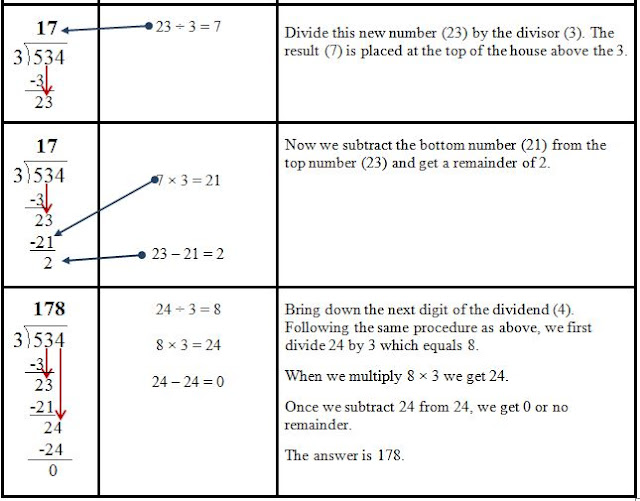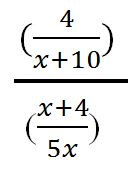### Ideas on How to Teach Long Division

As presented in an earlier posting, there is another way to approach long division.  However, since many of you are required to present it the long way, here are a couple of ideas to make it easier for your students.

First of all, have the students use graph paper.  The squares help to keep the numbers aligned which seems to be a problem for many students.  If you don't have graph paper, you can download free templates at Donna Young's Free Graph Paper and make your own.  I like the idea of separating the problems with lines to ensure there is no cross over from one problem to another.

Secondly, try using the acronym (a mnemonic device) of Does McDonald's Sell Cheese Burgers.  I have see this acronym many times on Pinterest, but usually the C is omitted.

Check means that after the student has subtracted, they should check to see if the remainder is smaller than the divisor.  If it is equal to or larger than the divisor, then enough was not taken out of the dividend.  This is a step often skipped when long division is taught; yet, if the student doesn't check and make the needed correction, the answer (quotient) will be wrong.

In order to learn division, the student must first have a good understanding of multiplication. Students don’t need to perfectly know all of the times tables, but a majority of the facts or having a reasonably quick strategy to figure out the answer is necessary.

Start by practicing division using the number series the students can easily skip count such as 2 and 5. Then gradually move up to nine. After that, move to division by double digit numbers using 10 since most students know how to skip count by 10. Once the concept is understood, teaching division will become more about guided practice to help your child to become comfortable with the division operation which, in reality, is a different kind of multiplication practice.

### The Long and Short of It - Two different ways to do long Division

My remedial college math class is currently working on fractions. (Yes, even many college students don't understand them!) When we discussed how to change an improper fraction to a mixed numeral, long division came up. I showed the class a shortcut I was taught many years ago (approximately when the earth was cooling) and none, no not even one student, had seen it before. I wonder how many of you are unfamiliar with it as well? First let's look at long division and how most students are taught today. We will use 534 divided by 3.Now if that doesn't make your head swim, I don't know what will. Everything written in the third column is what the student must mentally do to solve this problem. Then we wonder why students have trouble with this process. There is another way, and it is called short division for a reason. This is the way I learned it.......Only \$3.00
I don't know about you, but I would rather have my students doing mental math to solve division problems than writing everything out in the long form. And the paper and frustration you will save will be astounding! So what will it be.....long division or short division?

As a Side Note: Since many students do not know their multiplication tables, reducing fractions is almost an impossible task. The divisibility rules, if learned and understood, can be an excellent math
tool. This resource contains four easy to understand divisibility rules and includes the rules for 1, 5, and 10 as well as the digital root rules for 3, 6, and 9. A clarification of what digital root is and how to find it is explained. Also contained in the resource is a dividing check off list for use by the student. If you are interested, just click under the resource title page.

### Dividing Fractions Using KFC

Ugh - It's time to teach the division of fractions. My experience has been that many students forget which fraction to flip and often, they forget to change the dreaded division sign to a multiplication sign. The other evening,  I was helping my 5th grade granddaughter with her homework. Really, she had completed it by herself, but she wanted me to check it. At the top of her paper were the letters "KFC". I asked her what they meant, and she replied, "Kentucky Fried Chicken." Now I have taught math for years and years, and I had never heard of that one!

She explained that the "K" stood for keep; "F" for flip, and "C" for change. Let's suppose the problem on the left was one of the problems on her homework paper.First, she would Keep the first fraction. Next, she would Flip the second one, and then Change the division sign to a multiplication sign...like illustrated on the right. She would then cross cancel if possible (In this case it is).  Finally, she would multiply the numerator times the numerator and the denominator by the denominator to get the answer.
She was able to work all the division problems without any trouble by just remembering the letters KFC.Yesterday, I was working in our college math lab when a student needed help. On the right is the problem he was having difficulty with. (For those of you who don't teach algebra or just plain hate it, I am sure this problem looks daunting and intimidating. Believe me, my student felt the same way!)
First I had the student rewrite the problem with each fraction side by side with a division sign in between them like this.
Doesn't it look easier already? I then taught him KFC. You read that right! I did! (I figured if it worked for a 5th grader, it should work for him.) Surprisingly it made sense to him because he now had mnemonic device (an acronym) that he could easily recall. He rewrote the problem by Keeping the first fraction, Flipping the second, and Changing the division sign to a multiplication sign.
Now it was just a simple multiplication problem.  Had he been able to, he would have cross canceled, but in this case, he simply multiplied the numerator times the numerator and denominator by the denominator to get the answer.

So the next time you teach the division of fractions, or you come across a problem like the one above, don't panic!  Remember KFC, and try not to get hungry!

### Dump and Divide or Better Known As Converting Fractions to Decimals

When working with fractions, my remedial math college students are never quite sure which number to divide by. This same thing often occurred when I taught middle school and high school. So the question I had to answer was, "How can I help my students remember what number goes where?"

First, the student must understand and know the vocabulary for the three parts of a division problem. As seen in the problem above, each part is correctly named and identified.

Side Note: The symbol separating the dividend from the divisor in a long division problem is a straight vertical bar with an attached vinculum (you might have to look this word up) extending to the left, but it seems to have no established name of its own. Therefore, it can simply be called the "long division symbol" or the division bracket. I wish it were named something fancier, but sometimes plain and straight forward is the best!Now let's look at a fraction that the student is asked to rewrite as a decimal. The fraction on your right is two-fifths and is read from top to bottom as two divided by five. That's easy enough, but when my students enter this into their calculators, many will put in the 5 first, and then press the
division sign, followed by the 2. Of course, they get the wrong answer. Now let's look at the dump and divide method.

First, dump the 2 into the calculator. Then press the division sign; then divide by 5. The answer is 0.4.I am aware that many of students are not allowed to use calculators; so, let's look at how this method would work using the division bracket. We will use the same fraction of 2/5 and the same phrase, dump and divide.First, take the numerator and dump it inside the division bracket. (Note: Use N side instead of inside so that numerator and N side both start with "N".) Now place the 5 outside of the long division bracket and divide. The answer is still .4.

Dump and Divide will also work when a division problem is written horizontally as a number sentence such as: 15 ÷ 3. First, reading left to right, dump 15 into the division bracket. Now place the 3 on the outside. Ask, "How many groups of three are in 15?" The answer is 5.

Try using Dump and Divide with your students, and then let me know how it works. You can e-mail by clicking on the page entitled Contact Me or just leave a comment.

Something Else to Think About:  Since many students do not knowOnly \$3.00
their multiplication tables, reducing fractions is almost an impossible task. The divisibility rules, if learned and understood, can be an excellent math tool. The resource, Using Digital Root to Reduce Fractions, contains four easy to understand divisibility rules as well as the digital root rules for 3, 6, and 9. A clarification of what digital root is and how to find it is explained. Also contained in the resource is a dividing check off list for the student. Download the preview to view the first divisibility rule plus three samples from the student check off list.